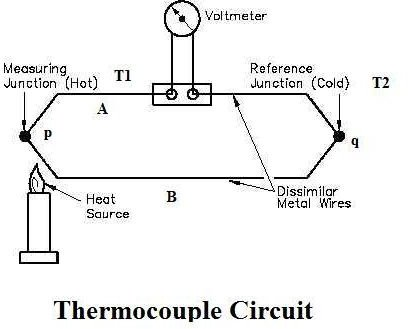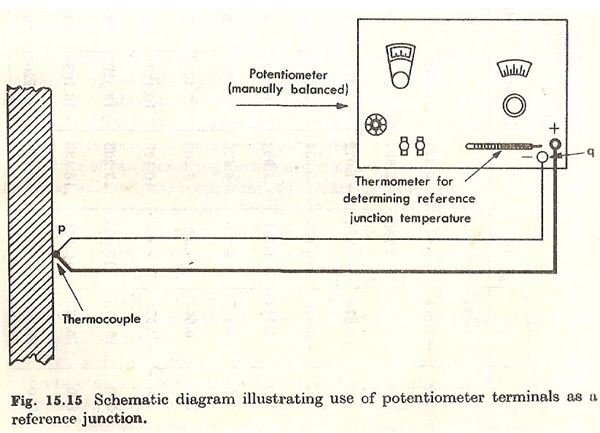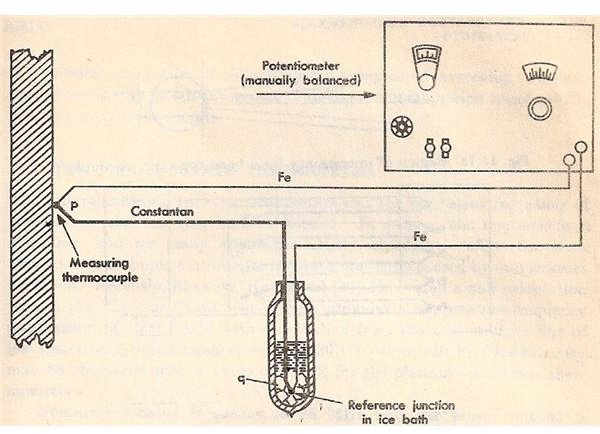Working Principle The working principle of thermocouple is based on three effects, discovered by Seebeck, Peltier and Thomson. They are as follows: 1) Seebeck effect: The Seebeck effect states that when two different or unlike metals are joined together at two junctions, an electromotive force (emf) is generated at the two junctions. The amount of emf generated is different for different combinations of the metals. 2) Peltier effect: As per the Peltier effect, when two dissimilar metals are joined together to form two junctions, emf is generated within the circuit due to the different temperatures of the two junctions of the circuit. 3) Thomson effect: As per the Thomson effect, when two unlike metals are joined together forming two junctions, the potential exists within the circuit due to temperature gradient along the entire length of the conductors within the circuit. In most of the cases the emf suggested by the Thomson effect is very small and it can be neglected by making proper selection of the metals. The Peltier effect plays a prominent role in the working principle of the thermocouple.

## Diagrams## How it Works

The general circuit for the working of thermocouple is shown in the figure 1 above. It comprises of two dissimilar metals, A and B. These are joined together to form two junctions, p and q, which are maintained at the temperatures T1 and T2 respectively. Remember that the thermocouple cannot be formed if there are not two junctions. Since the two junctions are maintained at different temperatures the Peltier emf is generated within the circuit and it is the function of the temperatures of two junctions. If the temperature of both the junctions is same, equal and opposite emf will be generated at both junctions and the net current flowing through the junction is zero. If the junctions are maintained at different temperatures, the emf’s will not become zero and there will be a net current flowing through the circuit. The total emf flowing through this circuit depends on the metals used within the circuit as well as the temperature of the two junctions. The total emf or the current flowing through the circuit can be measured easily by the suitable device.
Original Source]]>